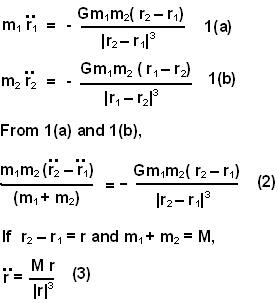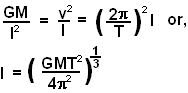## Monday, September 1, 2008

### Irodov Problem 1.205Since there is no net external force on the binary star system and so its CG will remain stationary. Suppose that r1 and r2 are the position vectors of the two stars at any given instant with CG as the origin and their masses be m1 and m2. Then we have,(3) is exactly the same as the equation of any body moving in a gravitational field due to a body of mass M.
In other words the distance vector connecting the stars will same as a Keplerian orbit. So in general the stars may not be at the same distance at all times. The problem however, asks for a constant distance. So for this problem let us assume that the planets are moving in a circular path around the CG. So from the point of view of a person on star 1 the other star will seem like it is going in a circle around it as if his star's mass is M.

The centripetal acceleration of the other star will be provided entire by this central force as seen in Eqn (3).

So if l is the distance between the two stars then we have,